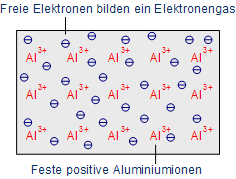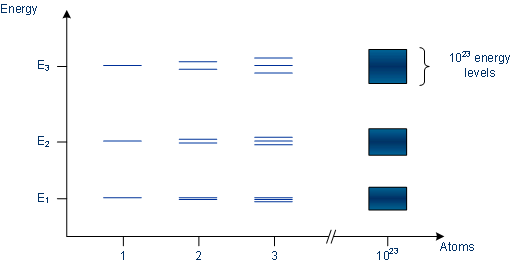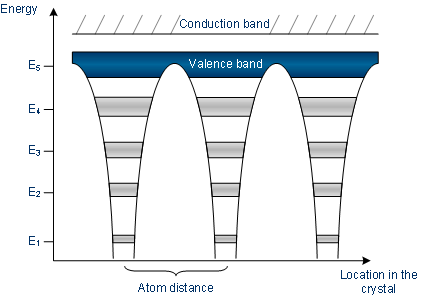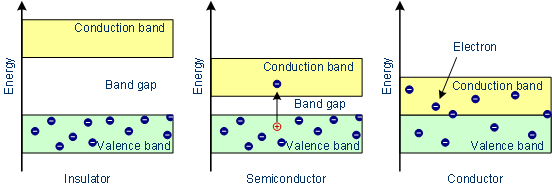# Semiconductor Technology from A to Z

## 1. Conductors

Conductors are generally substances which have the property to pass different types of energy. In the following, the conductivity of electricity is the value of interest.

### Metals

The conductivity of metals is based on the free electrons (so-called Fermi gas) due to the metal bonding. Already with low energy electrons become sufficiently detached from the atoms and a conductivity is achieved.

#### Metallic bonding: fixed ions and free valence electrons (Fermi gas)The conductivity depends, inter alia, on the temperature. If the temperature rises, the metal atoms swing ever stronger, so that the electrons are constrained in their movements. Consequence, the resistance increases. The best conductors, gold and silver, are used relatively rare because of the high costs (gold e.g. for the contacting of the finished chips). The alternatives in the semiconductor technology for the wiring of the individual components of microchips are aluminum and copper.

### Salts

In addition to metals, salts can also conduct electricity. There are no free electrons, so the conductivity depends on ions which can be solved when a salt is melting or dissolving, so that the ions are free to move (see chapter chemical bonds for details).

## 2. Insulators

Insulators possess no free charge carriers and thus are non-conductive.

### The atomic bond

The atomic bond is based on shared electron pairs of nonmetals. The elements which behave like nonmetals have the desire to catch electrons, thus there are no free electrons which might serve as charge carriers.

### The ionic bond

In the solid state, ions are arranged in a grid network. By electrical forces, the particles are held together. There are no free charge carriers to enable a current flow. Thus substances composed of ions can be both conductor and insulator.

## 3. Semiconductors

Semiconductors are solids whose conductivity lies between the conductivity of conductors and insulators. Due to exchange of electrons - to achieve the noble gas configuration - semiconductors arrange as lattice structure. Unlike metals, the conductivity increases with increasing temperature.

Increasing temperatures leads to broken bonds and free electrons are generated. At the location at which the electron was placed, a so-called defect electron ("hole") remains.

#### Cut-out of a silicon lattice

The electron flow is based on the conductivity properties of semiconductors. The electronic band structure illustrates why semiconductors behave like this.

## 4. The band model

The electronic band structure is an energy schema to describe the conductivity of conductors, insulators, and semiconductors. The schema consists of two energy bands (valence and conduction band) and the band gap. The valence electrons - which serve as charge carriers - are located in the valence band, in the ground state the conduction band is occupied with no electrons. Between the two energy bands there is the band gap, its width affects the conductivity of materials.

### The energy bands

If we consider a single atom, there are according to the Bohr model of atoms sharply distinct energy levels, which may be occupied by electrons. If there are multiple atoms side by side they are interdependent, the discrete energy levels are fanned out. In a silicon crystal, there are approximately 1023 atoms per cubic centimeter, so that the individual energy levels are no longer distinguishable from each other and thus form broad energy ranges.

#### Energy levels of atoms which are in interdependency with other atomsThe width of the energy bands depends on how strongly the electrons are bound to the atom. The valence electrons in the highest energy level interact strongly with those of neighboring atoms and can be solved relatively easily from an atom; with a very large number of atoms, a single electron can no longer be assigned to one single atom. As a result, the energy bands of the individual atoms merge to a continuous band, the valence band.

#### Energy bands of atoms which are in interdependency with other atoms### The band model of conductors

In conductors, the valence band is either not fully occupied with electrons, or the filled valence band overlaps with the empty conduction band. In general, both states occure at the same time, the electrons can therefore move inside the partially filled valence band or inside the two overlapping bands. In conductors there is no band gap between the valence band and conduction band.

### The band model of insulators

In insulators the valence band is fully occupied with electrons due to the covalent bonds. The electrons can not move because they're "locked up" between the atoms. To achieve a conductivity, electrons from the valence band have to move into the conduction band. This prevents the band gap, which lies in-between the valence band and conduction band.

Only with considerable energy expenditure (if at all possible) the band gap can be overcome; thus leading to a negligible conductivity.

### The band model of semiconductors

Even in semiconductors, there is a band gap, but compared to insulators it is so small that even at room temperature electrons from the valence band can be lifted into the conduction band. The electrons can move freely and act as charge carriers. In addition, each electron also leaves a hole in the valence band behind, which can be filled by other electrons in the valence band. Thus one gets wandering holes in the valence band, which can be viewed as positive charge carriers.

There are always pairs of electrons and holes, so that there are as many negative as positive charges, the semiconductor crystal as a whole is neutral. A pure undoped semiconductor is known as intrinsic semiconductor. Per cubic centimeter there are about 1010 free electrons and holes (at room temperature).

Since the electrons always assume the energetically lowest state, they fall back into the valence band and recombine with the holes if there is no energy supply. At a certain temperature an equilibrium is arranged between the electrons elevated to the conduction band and the electrons falling back. With increasing temperature the number of electrons that can leap the band gap is increased, and thus increasing the conductivity of semiconductors.

#### The band modelSince the width of the band gap represents a certain energy corresponding to a particular wavelength, one tries to alter the width selective in order to obtain certain colors of light emitting diodes (LED). This may be achieved by combining different materials. Gallium arsenide (GaAs) has a band gap of 1.4 eV (electron volts, at room temperature) and thus emits red light.

The intrinsic conductivity of silicon is of no interest for the functioning of components, since it depends, inter alia, on the supplied energy. Which means that it changes with the temperature; in addition a conductivity comparable to metals is only possible at very high temperatures (several hundred degrees Celsius). In order to deliberately influence the conductivity of semiconductors, impurity atoms can be introduced into the regular silicon lattice to alter the number of free electrons and holes.